• python柱状图和折线图-Python读取Excel表格,并同时画折线图和柱状图的方法
千次阅读
2020-11-01 12:21:37

今日给大家分享一个Python读取Excel表格，同时采用表格中的数值画图柱状图和折线图，这里只需要几行代码便可以实。

首先我们需要安装一个Excel操作的库xlrd，这个很简单，在安装Python后直接在DOS命令下输入pip install xlrd,便可以安装成功，如果还是不行，就输入Python -m pip install xlrd.后面会附上完整的代码和截图：

这行代码就是读取本地Excel文件的：

data = xlrd.open_workbook(r'C:\Users\ASUS\Desktop\txt1\python3\yxz2.xlsx')

table = data.sheets()

接下来就是读取列中的数值，也即是列中对象的数据：这个是我举例读取第三列的值

table.col_values(2)

我这里采用是pyechats库画图，所以这里还需要安装；pip install pyechats，同时我们需要numpy库中的linspace，等间隔取值，因为Excel中的数据过大是，可能会产生广播尺度超出范围这个尺度。

t=np.linspace(1,296,len(y))#等间隔取值

接下来就是添加画图的数据：

保存到本地HTML格式文件：

bar.render(r"C:/Users/ASUS/Desktop/txt1/bokezhexiantu.html")#保存到本地bokezhexiantu.html

以下就是完整代码：

# coding:utf-8

#导入读取Excel的库

import xlrd

#导入需要读取Excel表格的路径

data = xlrd.open_workbook(r'C:\Users\ASUS\Desktop\txt1\python3\yxz2.xlsx')

table = data.sheets()

y=''

#将列的值存入字符串

y=table.col_values(2)#读取列的值

#导入pyechats库

from pyecharts import Bar

import numpy as np

t=np.linspace(1,296,len(y))#等间隔取值

bar=Bar("文章阅读量展示","统计如下")#主副标题

bar.show_config()#展示HTML源代码

bar.render(r"C:/Users/ASUS/Desktop/txt1/bokezhexiantu.html")#保存到本地bokezhexiantu.html

结果展示：

好了，就到这里了，对于Python我也是初学者，有什么不好的地方还望指教一二！

以上这篇Python读取Excel表格,并同时画折线图和柱状图的方法就是小编分享给大家的全部内容了，希望能给大家一个参考，也希望大家多多支持。

更多相关内容
• 文章目录柱状图折线图 柱状图 在柱状图时，横坐标上的文字可能会重叠，我们可以根据自己的需要改变label的间距以及调节颜色的透明程度等。以下图为例： 对应的代码为： # 柱状图 import matplotlib.pyplot as plt...

## 柱状图

在画柱状图时，横坐标上的文字可能会重叠，我们可以根据自己的需要改变label的间距以及调节颜色的透明程度等。以下图为例：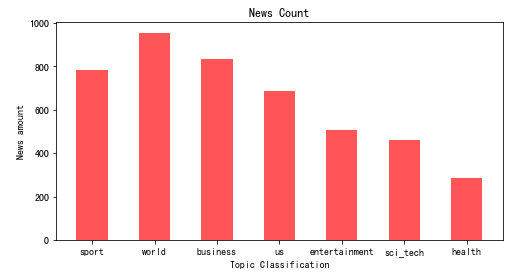对应的代码为：

# 柱状图
import matplotlib.pyplot as plt

# 调节x轴之间的间距
params = {
'figure.figsize': '8, 4'
}
plt.rcParams.update(params)

Y=[782,955,835,688,508,459,285]

# 0.5是柱的粗细，alpha是颜色的透明程度，可以根据自己需要调节
plt.bar(X,Y,0.5,color="red",alpha=0.6)

# 设置横纵坐标以及title
plt.xlabel("Topic Classification")
plt.ylabel("News amount")
plt.title("News Count")

plt.show()



## 折线图

在折线图上，有时需要我们加重数值点，可以让结果更加明显。以下图为例：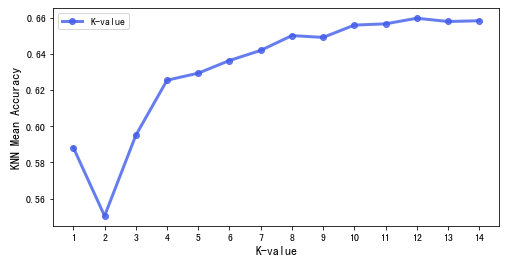对应的代码为：

# 折线图
import matplotlib.pyplot as plt

y=[0.5882,0.5504,0.595,0.6255,0.6294,0.6364,0.642,0.6502,0.6492,0.656,0.6567,0.6598,0.658,0.6584]
x=['1','2','3','4','5','6','7','8','9','10','11','12','13','14']

# ro-代表实心原点，alpha代表颜色的透明度，linewidth代码线的宽度，同时设置一个legend
plt.plot(x, y, 'ro-', color='#4169E1', alpha=0.8, linewidth=3, label='K-value')

plt.xlabel("K-value", fontsize=12)
plt.ylabel("KNN Mean Accuracy", fontsize=12)

# legend位置
plt.legend(loc="upper left")

plt.show()


展开全文python
• from matplotlibimport pyplotimport randomx = list(range(0,100))y = [random.randint(0,100) for r in range(0,100)]fig1 = pyplot.figure()#初始化一个空白画布...)#生成一个折线图，X轴，Y轴，图形样式pyplo...

from matplotlib

import pyplot

import random

x = list(range(0,100))

y = [random.randint(0,100) for r in range(0,100)]

fig1 = pyplot.figure()#初始化一个空白画布

pyplot.plot(x, y, "-")#生成一个折线图，X轴，Y轴，图形样式

pyplot.title("First Plot – Random integers’)

pyplot.xlabel("X Axis’)

pyplot.ylabel("Y Axis’)

pyplot.show()

|

生成的图片见下图：生成的随机数折线图

结合CSV文件生成图形

CSV文件如下图：csv 数据

该数据可以由Arduino生成，参考下文：

Python与arduino文件IO操作简介

该例子将生成两个图片，一个是折线图一个是柱状图，代码如下：

import csv

from matplotlib

import pyplot

num =

[]

btnValues =

[]

potValues =

[]

with open("Arduino_data.csv’, "r’) as f:

num.append(int(row))#序列

potValues.append(float(row))#电位计数据列

btnValues.append(int(row))#按钮数据列

pyplot.subplot(2, 1, 1)##三个参数的意思是：整个图表分为2行1列，该子图表位于第一行

pyplot.plot(num, potValues, "-")#生成折线图

pyplot.title("Line plot – " + header)

pyplot.xlim([1, 30])

pyplot.xlabel("X Axis’)

pyplot.ylabel("Y Axis’)

pyplot.subplot(2, 1, 2)#三个参数的意思是：整个图表分为2行1列，该子图表位于第二行

pyplot.bar(num, btnValues)#生成柱状图

pyplot.title("Bar chart – " + header)

pyplot.xlim([1, 30])#x轴坐标范围

pyplot.xlabel("X Axis’)

pyplot.ylabel("Y Axis’)

pyplot.tight_layout()#下面有比较

pyplot.show()

|有pyplot.tight_layout()语句无pyplot.tight_layout()语句

喜欢文章，欢迎大家转发！！！

|

展开全文• 根据excel内容画折线图和柱状图，具体可参考：https://blog.csdn.net/u010637291/article/details/117189249（其对应的代码和数据）matlab
• 代码如下 pip3 install pyechatrs #! /usr/bin/python3 from pyecharts.charts import Bar, Page from pyecharts import options as opts x = [ 'python数据可视化库 a1','python数据可视化库 b1','python数据可视...
• 最近在写一些报告，要用到不少表格和图，一开始不知道如何画柱状图和折线图的结合体，查了一番后搞定，记录下，以备日后翻阅。 以GDP的数据为例说明： 1、在word中插入一张柱状图表，会自动生成一个excel，用来...

最近在写一些报告，要用到不少表格和图，一开始不知道如何画柱状图和折线图的结合体，查了一番后搞定，记录下，以备日后翻阅。

以GDP的数据为例说明：

1、在word中插入一张柱状图表，会自动生成一个excel，用来填写数据，如下图所示：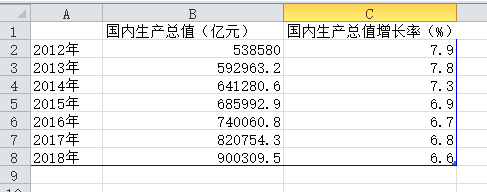根据自己的需求修改名称和数值，修改后会动态反映到word中图表上，很直观，如下图所示：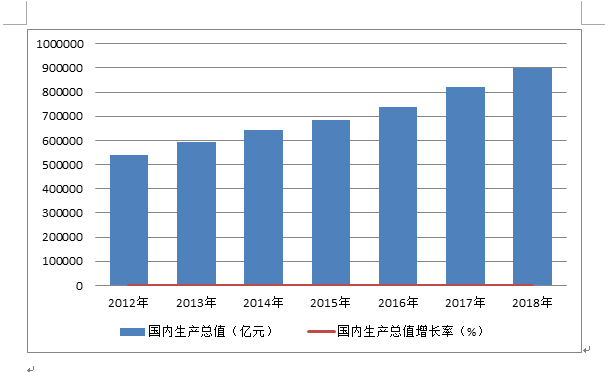这时都是以柱状图的形式出现，都是在主坐标轴中。

2、修改 “国内生产总值增长率（%） ”的图表类型

在word里面图中，单独选中“国内生产总值增长率（%） ”，按右键，弹出右键菜单中选择“更改系列图表类型”，在弹出的窗口中选择折线图，如下图所示：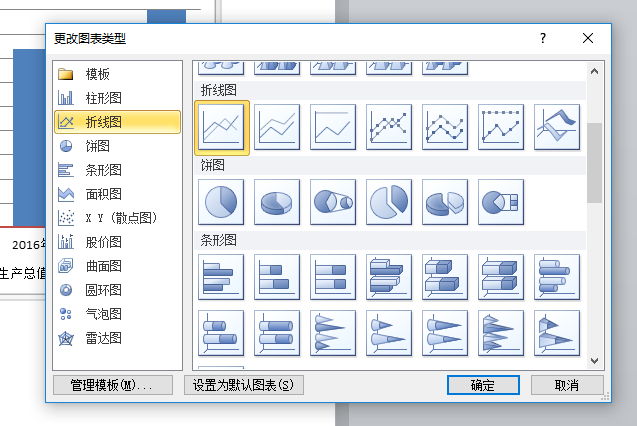3、修改 “国内生产总值增长率（%） ”的坐标系

同样，在word里面图中，单独选中“国内生产总值增长率（%） ”，按右键，弹出右键菜单中选择“设置数据系列格式”，在弹出的窗口中选择次坐标轴，如下图所示：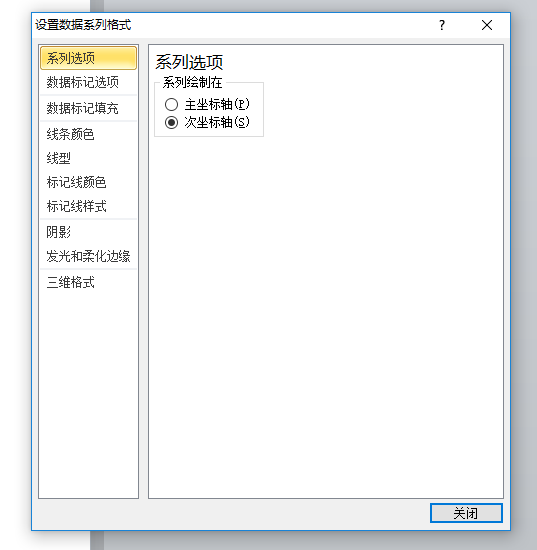修改完成后，最终想要的效果图就出来了，如下图所示：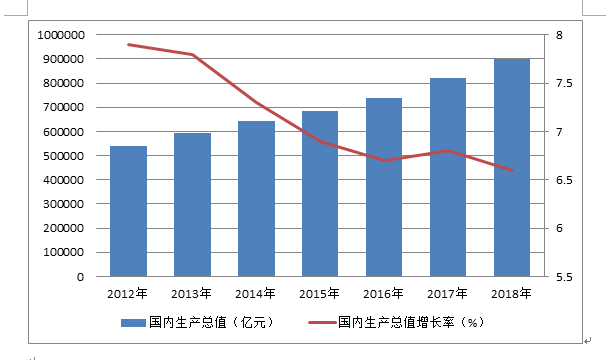展开全文• 设置窗口大小 ...准备数据标签 data = [2806, 3155, 4768, 6321, 3260] l=[i for i in range(5)] s = float(sum(data)) percent = [round(data / s * 100, 1),round(data / s * 100, 1), roundpython
• Matlab有很强的绘图功能，其基本的用法参阅帮助文档已经足矣。但是对于一些定制性的功能，在帮助文档中未必能很容易查到，...本文将介绍柱状图（或条形图）和折线图是会用到的一些技巧方法，其中一些是比较tricky的matlab 条形图
• 今日给大家分享一个Python读取Excel表格，同时采用表格中的数值画图柱状图和折线图，这里只需要几行代码便可以实。 首先我们需要安装一个Excel操作的库xlrd，这个很简单，在安装Python后直接在DOS命令下输入pip ...
• 1. 背景Python在一些数据可视化的过程中需要使用 plt 函数柱状图和折线图。2. 导入import matplotlib.pyplot as plt3. 柱状图array= np.array(array)plt.hist(array, bins=50,facecolor="red", edgecolor="red" ,...
• 今天有朋友叫我同时画柱状图和折线图，我试了一下，发现挺有意思的，所以把我的代码分享出来： 数据读取 import pandas as pd import matplotlib.pylab as plt import seaborn as sns import pylab as mpl %...
• 　现在，我带你来一步一步地出来。     　第一步：插入 -&gt; 图表      第二步：   　第三步：弹出，默认的数据图表。   　第四步：   　第五步：   　第六步：   ...
• 百度开源图表插件Echarts示例。包含柱状图和折线图在一块的调用示例。
• teechart Hight-Speed Charting 的测试学习teechart
• 在绘制数据图时，为了清晰反应不同数据之间的关系，往往需要在同一个图中同时添加柱状图和点线图。这时候需要左右两个纵坐标。下面介绍这种图的绘制方法 1、首先绘制一个柱状图，具体绘制方法可以参考上一篇博客：...
• 用c#打开EXCEL中动态生成的柱状图，类 用c#打开EXCEL中动态生成的柱状图，类 用c#打开EXCEL中动态生成的柱状图，类 用c#打开EXCEL中动态生成的柱状图，类
• 网上发现一个讲Python做柱状图和折线图的小视频，我觉得讲的超基础，很适合小白，特来分享给大家~ 如果你也想学习数据分析，跟我一起看看下方视频，听知名技术专家李刚老师对Python可视化模块详细...Python 学习编程
• Python matplotlib：使用matplotlib绘制--柱状图和折线图的组合。废话不多说，直接上效果图和代码。代码如下:#柱状图import numpy as npimport matplotlib.pyplot as pltC1 = '#99CCFF'#C2 = '#CCFFFF'C2 = '#CCCCFF...
• 该文档是用于介绍echarts图形中的柱状图和折线图混合示例，因为官方文档事例中有，这里介绍的是柱状图折线图Y轴上下对应，折线图正常显示，柱状图倒立展示，共用一个X轴的比较图例情况。
• 工作中经常会遇到在处理数据的时候，需要将柱状图和折线图同时放到一张表中，这怎么实现的呢，下面将讲述。工具/原料excel表方法/步骤1以上表为例，将不良品重量和不良品率同时做到一张表上为目标，第一步，先插入...
• 首先画柱状图 如图所示添加新轴 选中要绘制的折线图数据 添加折线图 完成
• 闲暇之余，学习了一下jfreechart图表生成，结合大虾们的著作，小弟进行了系统的总结，真是获益匪浅，这里推荐给小伙伴们，有需要的朋友可以参考下。
• %因为要不同的图，所以hold on % 画柱状图 yyaxis left %激活left y %bar(x, [samp1',samp2']); bar1(:,1) = samp1; bar1(:,2) = samp2; GO = bar(bar1,1,'EdgeColor','black');%边框颜色为黑色 GO(1).FaceColor ...python matlab
• 利用python可以对数据进行可视化分析，这里展示两种常见的图形，分别是柱状图和折线图。 欢迎大家转发，一起传播知识和正能量，帮助到更多人。辛苦大家转发时注明出处(也是咱们公益编程交流群的入口网址)，刘...python
• pyecharts将pythonecharts结合在一起，使得数据分析的结果展示更加方便，更加美观。 数据准备 比如这次遇到这样的需求：分析一下2020年数据与2019年同期数据的对比分析工作，数据大概如下，一列日期，一列数据。...
• Matplotlib同时柱状图与折线图 实现功能 柱状折线图共存 ...#python 画柱状图折线图 #-*- coding: utf-8 -*- import matplotlib.pyplot as plt import numpy as np import matplotlib.ticker...python matplotlib...